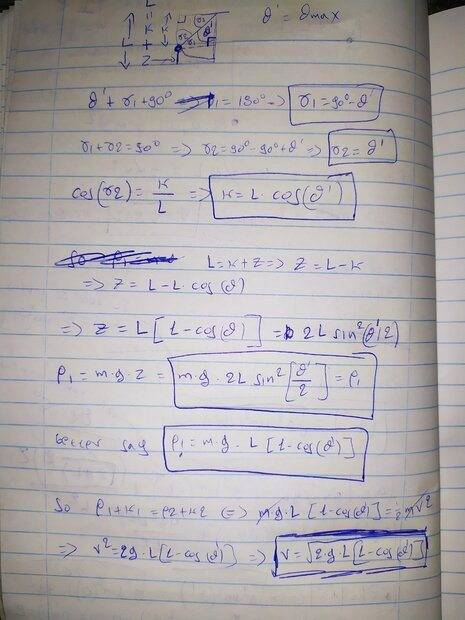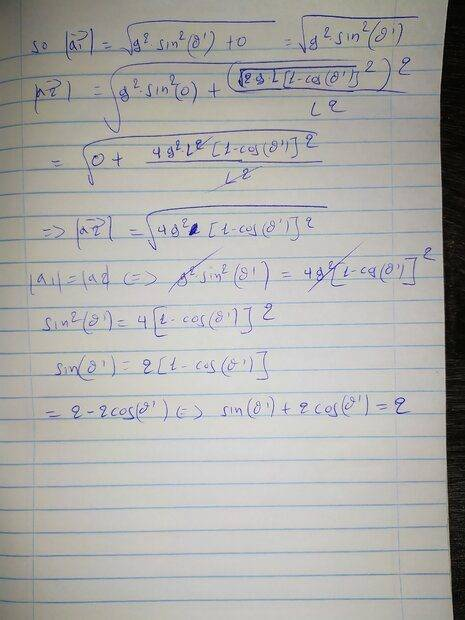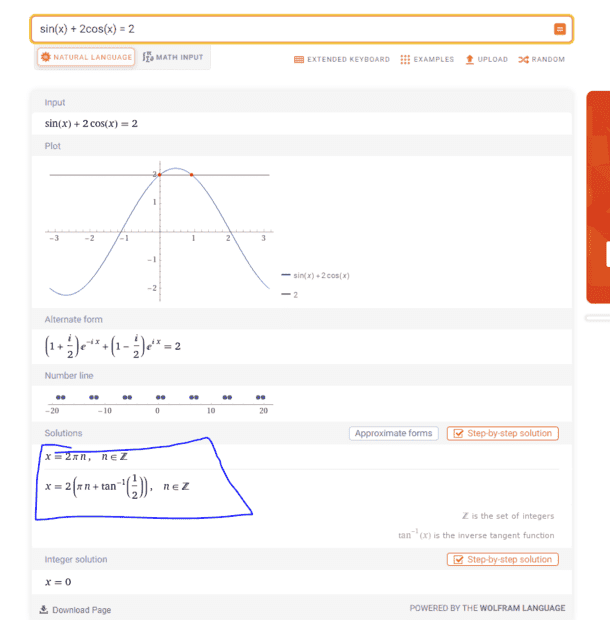# For a Pendulum: Knowing Acceleration Find Maximum Angle

• babaliaris
In summary, the pendulum in a clock goes up and down (oscillates) at the same speed, meaning that when the pendulum reaches its maximum height it changes direction instantly.

#### babaliaris

PS: By the way today I had exams in Physics and this problem was the first one I had to solve :p (unlucky) The question was to find the maximum angle θ that the pendulum can reach if we know that the magnitude of the acceleration is the same when the mass is located in the highest and the lowest point. The lowest point is when the mass passes exactly from the vertical position and the highest is when the angle θ(t) becomes maximum (which means that |V|=0).

And the problem is, if V does not change in speed, how can it become zero? How can the pendulum oscillate without the speed ever becoming zero at the maximum angle?

I will attach my solution which is exactly what I wrote in the exams today.

The problem description goes as follows:
"An object of mass m hangs from the roof with a rope of length L. The maximum angle that is between the rope and the vertical line (the one that is perpendicular to the roof where the rope is attached) is Θ. The magnitude of the acceleration is the same at the highest and lowest point of the path. Find θ in radians."

My solution, in short, was the following (note that when I say Θ(t) I don't mean the maximum angle, I just mean the function over time of the angle. in other words Θ = max{Θ(t)}):

Using everything I learned from this post, I found that
##a_{T} = gsin(θ(t))##
Also, I know ##a_{N} = \frac{|V|^2}{L}##
and then I found out that ##|a| = \sqrt{a_{T}^{2} + a_{N}^{2}} = \sqrt{g^{2}sin^{2}(θ(t)) + \frac{|V|^4}{L^{2}}}##

and then I said that ##|a1|= \sqrt{g^{2}sin^{2}(θ(t)) + 0} = gsin(θ(t)) ## since at the highest point the speed is zero and ##|a2| = \sqrt{g^{2}sin^{2}(θ(t)) + \frac{ |V_{max}|^4 }{L^2} }##

and if you say ##|a1| = |a2| <=>gsin(θ(t)) =\sqrt{g^{2}sin^{2}(θ(t)) + \frac{ |V_{max}|^4 }{L^2} } <=> g^{2}sin^{2}(θ(t)) = g^{2}sin^{2}(θ(t)) + \frac{|V_{max}|^4}{L^2} => V_{max} = 0## which is not correct.

#### Attachments

• Exams Problem Pendulum.pdf
507.3 KB · Views: 59
Last edited:
PS: O MY GOD, I just figured something out... Well if you see the pendulum in a clock, you see it going up and down (oscillating) at the same speed! This means that when the pendulum reaches its maximum height it changes direction instantly! This is why the speed never changes but the direction of V changes!

This is the wrong assumption that I did and used V = 0 at the highest point which is not correct!

I did what my tutor always told me not to do "Do not assume things based on your intuition. Only rely on the math and the data that was given".

But now, how can I use the equation ##|a| = \sqrt{a_{T}^{2} + a_{N}^{2}} = \sqrt{g^{2}sin^{2}(θ(t)) + \frac{|V|^4}{L^{2}}}## and that |a1|=|a2| (1 = highest point in the path, 2 = lowest point)?

By the way, since this path is an arc, then ##|V| = ωL##

babaliaris said:
PS: O MY GOD, I just figured something out... Well if you see the pendulum in a clock, you see it going up and down (oscillating) at the same speed! This means that when the pendulum reaches its maximum height it changes direction instantly! This is why the speed never changes but the direction of V changes!

This is the wrong assumption that I did and used V = 0 at the highest point which is not correct!
You've gone off the rails here. The speed is not constant and the speed is zero at the maximum height.

babaliaris said:
And the problem is, if V does not change in speed, how can it become zero? How can the pendulum oscillate without the speed ever becoming zero at the maximum angle?
The speed is zero but the acceleration is nonzero.

babaliaris said:
Using everything I learned from this post, I found that [...]
This is not the correct way to explain what you're doing. You need to provide the details as you go, just like textbook authors do when they solve worked examples.

•Lnewqban
My solution is in the pdf I attached. I don't know how to write everything in latex this is why I uploaded the pdf.

It is not an SHM problem. Since the magnitude of the angle is initially unknown, you cannot assume the small angle approximation.
Just consider the two positions, maximum amplitude and zero amplitude.
In terms of ##\theta_{max}##, what is the linear acceleration at that angle (magnitude and direction)?
In terms of ##v_{max}##, what is the linear acceleration at zero angle (magnitude and direction)?
What equation relates ##\theta_{max}## and ##v_{max}##?

•PeroK
babaliaris said:
PS: O MY GOD, I just figured something out... Well if you see the pendulum in a clock, you see it going up and down (oscillating) at the same speed! This means that when the pendulum reaches its maximum height it changes direction instantly! This is why the speed never changes but the direction of V changes!
Is that what you see in the animation of post #21 of your previous thread?

https://www.physicsforums.com/threa...rce-how-to-calculate-the-full-vector.1045791/

I respectfully disagree with your teacher, evidently, you can describe it with math, but have not been able to see how it happens in real life.•babaliaris
haruspex said:
It is not an SHM problem. Since the magnitude of the angle is initially unknown, you cannot assume the small angle approximation.
Just consider the two positions, maximum amplitude and zero amplitude.
In terms of ##\theta_{max}##, what is the linear acceleration at that angle (magnitude and direction)?
In terms of ##v_{max}##, what is the linear acceleration at zero angle (magnitude and direction)?
What equation relates ##\theta_{max}## and ##v_{max}##?
Why are you ignoring the centripetal acceleration?

•babaliaris
babaliaris said:
But now, how can I use the equation ##|a| = \sqrt{a_{T}^{2} + a_{N}^{2}} = \sqrt{g^{2}sin^{2}(θ(t)) + \frac{|V|^4}{L^{2}}}## and that |a1|=|a2| (1 = highest point in the path, 2 = lowest point)?

By the way, since this path is an arc, then ##|V| = ωL##
The fundamental observations you are missing are:

1) At the highest point, the bob is instantaneously at rest. The acceleration, therefore, is entirely tangential to the path. Otherwise, the bob would leave the circular path as it starts its downward swing. In other words, there is no centripetal acceleration at this point, owing to its zero velocity.

2) At the lowest point, the only forces (tension and gravity) are vertical, so there is no tangential acceleration. The acceleration at this point is entirely centripetal.

•Lnewqban and babaliaris
I thought of something else but I got a math error:

V at the lowest point:
##p1+k1 = p2 + k2 <=> mgL + 0 = 0 + \frac{1}{2}mV^2 <=> v = \sqrt{2gL}##

So at the highest point I now that: ##V = 0##, ##Θ(t) = Θ' = max{Θ(t)}##
and for the lowest point I know that: ##Θ(t) = 0## and ##v = \sqrt{2gL}##

and knowing from my prev calculations that ##|a| = \sqrt{g^2 sin^2 (θ(t)) + \frac{ (|V|^2)^2}{L^2}}##

so ##|a1| = \sqrt{ g^2 sin^2 (Θ') + 0 }##
##|a2| = \sqrt{ g^2 sin^2 (0) + \frac{ (\sqrt{2gL}^2)^2}{L^2} }##
##|a2| = \sqrt{ g^2 sin^2 (0) + \frac{ 4g^2L^2}{L^2} }##
##|a2| = \sqrt{ g^2 sin^2 (0) +4g^2 }##
##|a2| = \sqrt{ 4g^2 }##

so ##|a1|=|a2| <=> \sqrt{ g^2 sin^2 (Θ')} = \sqrt{ 4g^2 } <=> g^2 sin^2 (Θ') = 4g^2##
##<=>sin^2 (Θ') = 4 <=> sin(Θ') = 2 <=>Θ' = sin^{-1}(2) ##

but ##sin^{-1}(x)## is defined only for ##|x| < 1## i believe. The calculator gives an error for ##sin^{-1}(2)##

•PeroK
PeroK said:
@babaliaris not the easiest pendulum problem!
Off Topic: Yes. By the way, this is first-year university physics. There were so many students that were cheating in the past, so the professor snapped and started creating some really difficult problems. This is actually one of the easiest problems he had in exams. I'm a fifth year and I know calc 1, 2, and complex analysis and still, I can't solve his problems. First-year students are probably depressed by now :p 90% of them fail with 2-3 out of 10 (marks).

babaliaris said:
##p1+k1 = p2 + k2 <=> mgL + 0 = 0 + \frac{1}{2}mV^2 <=> v = \sqrt{2gL}##
How do you get mgL for the PE at the highest point it reaches, ##\theta_{max}##?

•babaliaris
I thought it didn't matter, so I assumed θ=90 where the m mass is indeed L meters from the ground.
So this is a mistake?

Yes at θmax I don't know the actual height so I can't find the potential energy there.

So in order for this to be right, I have to say:
##mgz = \frac{1}{2}mV^2## where z is the height when the pendulum is at ##Θ_{max}##?

babaliaris said:
I thought of something else but I got a math error:

V at the lowest point:
##p1+k1 = p2 + k2 <=> mgL + 0 = 0 + \frac{1}{2}mV^2 <=> v = \sqrt{2gL}##

So at the highest point I now that: ##V = 0##, ##Θ(t) = Θ' = max{Θ(t)}##
and for the lowest point I know that: ##Θ(t) = 0## and ##v = \sqrt{2gL}##

and knowing from my prev calculations that ##|a| = \sqrt{g^2 sin^2 (θ(t)) + \frac{ (|V|^2)^2}{L^2}}##

so ##|a1| = \sqrt{ g^2 sin^2 (Θ') + 0 }##
##|a2| = \sqrt{ g^2 sin^2 (0) + \frac{ (\sqrt{2gL}^2)^2}{L^2} }##
##|a2| = \sqrt{ g^2 sin^2 (0) + \frac{ 4g^2L^2}{L^2} }##
##|a2| = \sqrt{ g^2 sin^2 (0) +4g^2 }##
##|a2| = \sqrt{ 4g^2 }##

so ##|a1|=|a2| <=> \sqrt{ g^2 sin^2 (Θ')} = \sqrt{ 4g^2 } <=> g^2 sin^2 (Θ') = 4g^2##
##<=>sin^2 (Θ') = 4 <=> sin(Θ') = 2 <=>Θ' = sin^{-1}(2) ##

but ##sin^{-1}(x)## is defined only for ##|x| < 1## i believe. The calculator gives an error for ##sin^{-1}(2)##
You are confusing this problem with the earlier one. In that problem the pendulum starts at the horizontal position and the initial potential energy is ##U=mgL.## Here, the pendulum starts at angle ##\theta_{\text{max}}.## What is the potential energy?

On edit: I see that @haruspex already asked the same question. Draw a triangle and find the height. It's the length ##L## minus the vertical length from the support point to the mass.

•babaliaris
kuruman said:
Here, the pendulum starts at angle ##\theta_{\text{max}}.## What is the potential energy?
##P_{@Θ_{max}} = mgz## where z = the distance from the ground up to the mass m, and z is uknown...

babaliaris said:
##P_{@Θ_{max}} = mgz## where z = the distance from the ground up to the mass m, and z is uknown...
See my edited post about finding the height.

•babaliaris
Found it ##z = L[1-cosθ']## where ##θ' = θ_{max}##I ended up to ##sinθ' + 2cos(θ') = 2##. Unfortunately, I don't remember how to solve that. I always forget trig formulas...

Edited:Last edited:
Use the trig identities of the half angle in the second equation from the bottom
##\sin\theta=2\sin(\frac{\theta}{2})\cos(\frac{\theta}{2})##
##1-\cos\theta=2\sin^2(\frac{\theta}{2})##

•babaliaris
Or, just square the equation$$\sin \theta = 2(1-\cos \theta)$$ and use ##\sin^2 \theta + \cos^2 \theta = 1##. That's one formula you should never forget!

•Lnewqban
So in conclusion, Is this the correct solution? Did I make it!? Thank you for your time and guidance!

babaliaris said:
So in conclusion, Is this the correct solution? Did I make it!? Thank you for your time and guidance!
What solution? Note that ##\theta = 0## is a degenerate solution.

•babaliaris
All the math and physics that I did to reach in the equation ##sinθ' + 2cos(θ') = 2##

babaliaris said:
All the math and physics that I did to reach in the equation ##sinθ' + 2cos(θ') = 2##
That's correct, but it's not what I'd call a solution.

•babaliaris
babaliaris said:
All the math and physics that I did to reach in the equation ##sinθ' + 2cos(θ') = 2##
You still have to solve it and find a numerical value for ##\theta_{\text{max}}.##

Actually, this is a nice problem. It's the first time I see it.

•PeroK and babaliaris
I will do it tomorrow since I'm exhausted. I'm struggling with that problem for 3 days now. Never in my life, I had to deal with a problem for 3 days continuously. I'm glad that I finally figured it out!

How did great scientists try to solve a problem for years and not get crazy?

With the benefit of hindsight there is an easier way to solve this.
Just recognize it's a 3-4-5 triangle with ##\cos\theta=\dfrac{3}{5}~;~\sin\theta=\dfrac{4}{5}.##

•Lnewqban